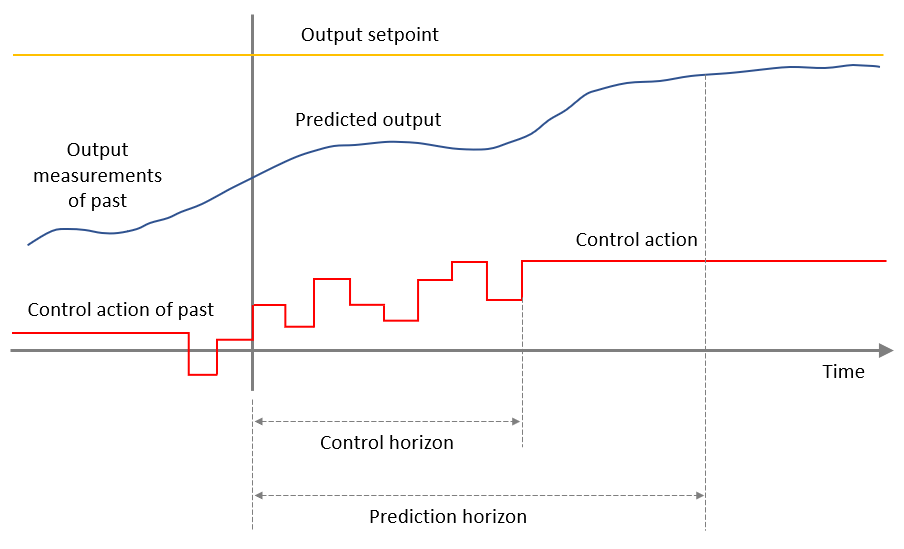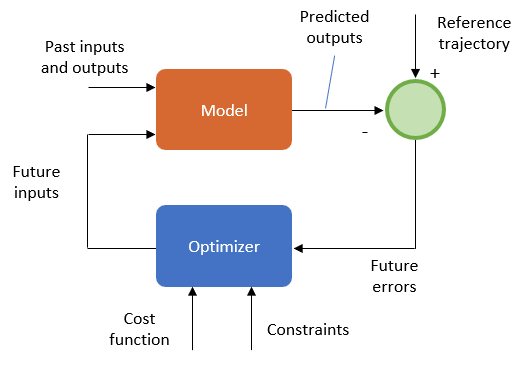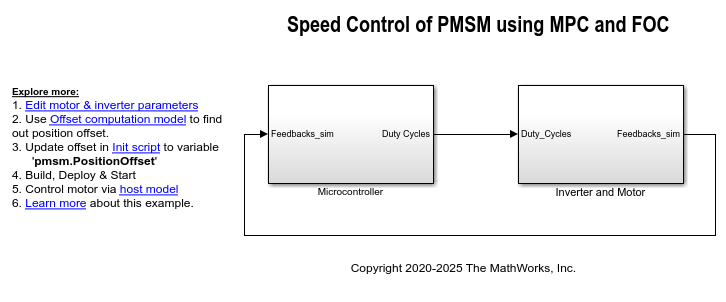# Run Field Oriented Control of PMSM Using Model Predictive Control

This example uses Model Predictive Control (MPC) to control the speed of a three-phase permanent magnet synchronous motor (PMSM). MPC is a control technique that tunes and optimizes the inputs to a control system to minimize the error in the predicted system output and achieve the reference control objective over a period of time. This technique involves solving the objective function and finding an optimal input sequence at every sample time (). After each time step, the current state of the plant is considered as the initial state and the above process is repeated.The optimizer provides the optimal inputs to the model based on solving the objective function under specific bounds and constraints. During Prediction step, the future response of a plant is predicted with the help of a dynamic discrete-time model up to Np sampling intervals, which is called the prediction horizon. During Optimization step, the objective function is solved to obtain the optimal control inputs up to Nc sampling intervals, which is called control horizon for the predicted response. Control horizon remains less than or equal to the prediction horizon.The example uses an MPC controller as a current controller (in a field-oriented control or FOC algorithm) to optimize theandcurrents and change the d-axis and q-axis controller voltage outputs so that they meet the reference control objectives over a period of time.

The objective function is derived as a linear sum of these:

[W1 * (error in output)] + [W2 * (rate of change of input)] + [W3 * (error in input)]

where, W1, W2, and W3 are the weightages.

The example uses the model initialization script to define these weightage (or weights) of these three parameters.

1. Inputs:2. Rate of change of input:3. Measured Outputs:Therefore, by default, the example gives maximum weightage to the output variables parameter (corresponding toandvoltages) when calculating the error in the predicted output. You can change the weightage values for error computation using the model initialization script available in the example.

The example also operates the MPC inputs (and) and the MPC outputs (and) under the following lower and upper bounds:

• Inputs• Measured outputsNote: The rate of change of input does not have any lower and upper bounds.

To retain linearity of the constraints, you can consider polytopic approximations. An acceptable trade-off between the accuracy and number of constraints can be acheived by approximating the feasible region using a hexagon. Because the direct component of the stator currentis almost always very close to zero, except during flux weakening operation when it takes negative values, you can consider the constraintis less that or equal to 0, to reduce the number of constraints.

The following image shows the pictorial representation of the contraints for the MPC output voltages (and, with circle approximation having 6 faces), and MPC input currents (and, with half-circle approximation having 4 faces). You can generate these plots by using the MATLAB command mcb_getMPCObject(pmsm,PU_System,Ts_current,T_pwm,1).Note: The sample time () used in the model intitialization script of this example is based on tests on the particular hardware. You can change the sample time for a differet kind of hardware, which will in turn impact the MPC operation.

### Models

The example includes the model mcb_pmsm_foc_mpc_qep_f28379d

You can use these models for both simulation and code generation. You can also use the open_system command to open the Simulink® models. For example, use this command for a F28379d based controller.

open_system('mcb_pmsm_foc_mpc_qep_f28379d.slx');For the model names that you can use for different hardware configurations, see the Required Hardware topic in the Generate Code and Deploy Model to Target Hardware section.

### Required MathWorks® Products

To simulate model:

• Motor Control Blockset™

• Model Predictive Control Toolbox™

To generate code and deploy model:

• Motor Control Blockset™

• Model Predictive Control Toolbox™

• Embedded Coder®

• Embedded Coder® Support Package for Texas Instruments™ C2000™ Processors

### Prerequisites

1. Obtain the motor parameters. We provide default motor parameters with the Simulink® model that you can replace with the values from either the motor datasheet or other sources.

However, if you have the motor control hardware, you can estimate the parameters for the motor that you want to use, by using the Motor Control Blockset parameter estimation tool. For instructions, see Estimate PMSM Parameters Using Recommended Hardware.

The parameter estimation tool updates the motorParam variable (in the MATLAB® workspace) with the estimated motor parameters.

2. If you obtain the motor parameters from the datasheet or other sources, update the motor parameters and inverter parameters in the model initialization script associated with the Simulink® models. For instructions, see Estimate Control Gains and Use Utility Functions.

If you use the parameter estimation tool, you can update the inverter parameters, but do not update the motor parameters in the model initialization script. The script automatically extracts motor parameters from the updated motorParam workspace variable.

### Simulate Model

This example supports simulation. Follow these steps to simulate the model.

1. Open a model included with this example.

2. Click Run on the Simulation tab to simulate the model.

3. Click Data Inspector on the Simulation tab to view and analyze the simulation results.

### Generate Code and Deploy Model to Target Hardware

This section instructs you to generate code and run the FOC algorithm on the target hardware.

This example uses a host and a target model. The host model is a user interface to the controller hardware board. You can run the host model on the host computer. The prerequisite to use the host model is to deploy the target model to the controller hardware board. The host model uses serial communication to command the target Simulink® model and run the motor in a closed-loop control.

Required Hardware

This example supports this hardware configuration. You can also use the target model name to open the model for the corresponding hardware configuration, from the MATLAB® command prompt.

For connections related to the preceding hardware configurations, see LAUNCHXL-F28069M and LAUNCHXL-F28379D Configurations.

Generate Code and Run Model on Target Hardware

1. Simulate the target model and observe the simulation results.

2. Complete the hardware connections.

3. The model automatically computes the ADC (or current) offset values. To disable this functionality (enabled by default), update the value 0 to the variable inverter.ADCOffsetCalibEnable in the model initialization script.

Alternatively, you can compute the ADC offset values and update it manually in the model initialization scripts. For instructions, see Run 3-Phase AC Motors in Open-Loop Control and Calibrate ADC Offset.

4. Compute the quadrature encoder index offset value and update it in the model initialization scripts associated with the target model. For instructions, see Quadrature Encoder Offset Calibration for PMSM Motor.

NOTE: Verify the number of slits available in the quadrature encoder sensor attached to your motor. Check and update the variable pmsm.QEPSlits available in the model initialization script. This variable corresponds to the Encoder slits parameter of the quadrature encoder block. For more details about the Encoder slits and Encoder counts per slit parameters, see Quadrature Decoder.

5. Open the target model for the hardware configuration that you want to use. If you want to change the default hardware configuration settings for the model, see Model Configuration Parameters.

6. Load a sample program to CPU2 of LAUNCHXL-F28379D, for example, program that operates the CPU2 blue LED by using GPIO31 (c28379D_cpu2_blink.slx), to ensure that CPU2 is not mistakenly configured to use the board peripherals intended for CPU1.

7. Click Build, Deploy & Start on the Hardware tab to deploy the target model to the hardware.

8. Click the host model hyperlink in the target model to open the associated host model. You can also use the open_system command to open the host model. For example, use this command for a F28069M based controller.

open_system('mcb_pmsm_foc_host_model_f28379d.slx');For details about the serial communication between the host and target models, see Host-Target Communication.

9. In the host model, open the blocks Host Serial Setup, Host Serial Receive, and Host Serial Transmit, and select a Port.

10. Update the Reference Speed value in the host model.

11. Click Run on the Simulation tab to run the host model.

12. Change the position of the Start / Stop Motor switch to On, to start running the motor.

13. Use the Debug signals section to select the debug signals that you want to monitor. Observe the debug signals from the RX subsystem, in the Time Scope of the host model.

### References

• G. Cimini, D. Bernardini, A. Bemporad and S. Levijoki, "Online model predictive torque control for Permanent Magnet Synchronous Motors," 2015 IEEE International Conference on Industrial Technology (ICIT), 2015, pp. 2308-2313, doi: 10.1109/ICIT.2015.7125438.

• S. Chai, L. Wang and E. Rogers, "Cascade model predictive control of a PMSM with periodic disturbance rejection," 2011 Australian Control Conference, 2011, pp. 309-314.